# Multiple Examples to Understand Sequences in Oracle

## Multiple Examples to Understand Sequences in Oracle

In this article, I am going to discuss Multiple Examples to Understand Sequences in Oracle. Please read our previous article where we discussed the basic concepts of Sequences in Oracle.

##### Examples of Sequences in Oracle

In our previous article, we created a sequence DEPT_S and we also created a table DEPT_TEST_S. We have inserted two values into the table and checked the sequences. Now, we will try to learn more about sequences. Let’s create another sequence DEPT_S1.

##### Example 1:

We are creating the sequence DEPT_S1 which will start with 10 as the current value and the next value will be incremented by 20. Let’s create the sequence.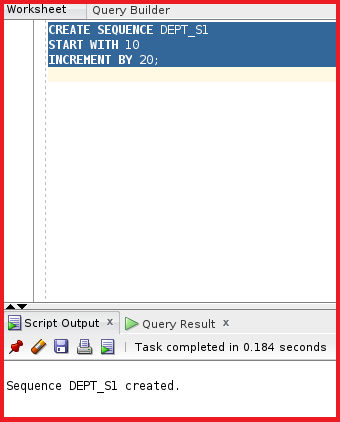So, the sequence DEPT_S1 is created. Let’s check the sequence details by using the data dictionary USER_SEQUENCES.

select * from USER_SEQUENCES where SEQUENCE_NAME =’DEPT_S1’;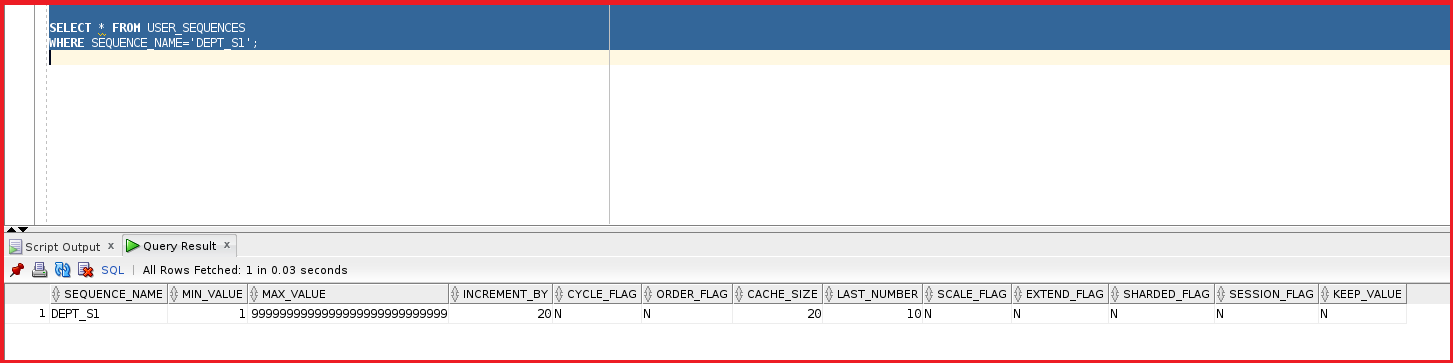So, we can see the details of the sequences with minimum value as 1 and increment_by is 20. The last number is 10. Now, let’s delete the data in the table DEPT_TEST_S.

DELETE FROM DEPT_TEST_S;

We are just deleting the data into the table DEPT_TEST_S.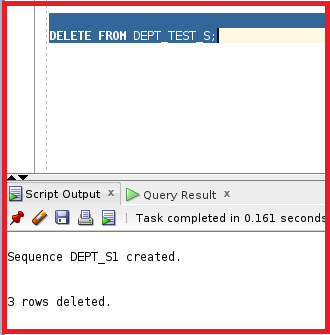So, the data inside the table DEPT_TEST_S is deleted. Let’s now try to insert new records in the table DEPT_TEST_S.

```INSERT INTO DEPT_TEST_S (DEPNO, DNAME) VALUES (DEPT_S1.NEXTVAL,'Marketing');
INSERT INTO DEPT_TEST_S (DEPNO, DNAME) VALUES (DEPT_S1.NEXTVAL,'Help Desk');```

So, we are inserting the DNAME as Marketing and Help Desk. The DEPNO will be the next value of the sequences. The current value of the sequences is 10 and the next value is incremented by 20. So, the next value will be 30. Let’s try to insert the data into the table DEPT_TEST_S.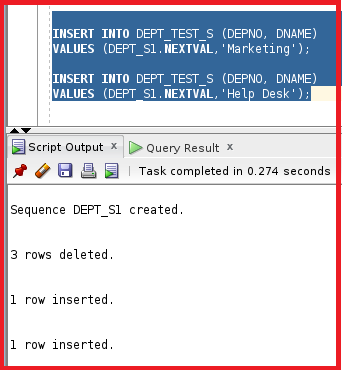So, the data is inserted into the table DEPT_TEST_S. Let’s now check the table to see the data and the sequence value.

select * from DEPT_TEST_S;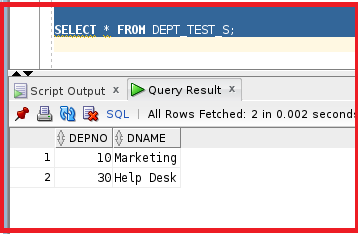We can see the depno is 10 for the first record and for the second record, the depno value is 30. This is because we have mentioned the sequence starts with 10 and increments by 20. So, if we add another record into the table then the DEPNO value will be 50. Let’s drop this data and drop the sequence.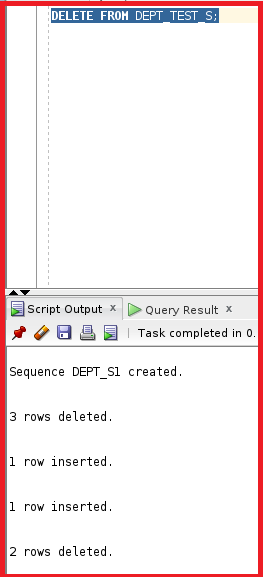So, the data inside the table DEPT_TEST_S is deleted.

##### Example 2:

Let’s have a look at another example where the sequences will be mentioned in negative.

CREATE SEQUENCE DEPT_S2 INCREMENT BY -5;

We are creating a sequence DEPT_S2 which will be incremented by -5. We haven’t mentioned the “Starts with”. Let’s go ahead and create the sequence.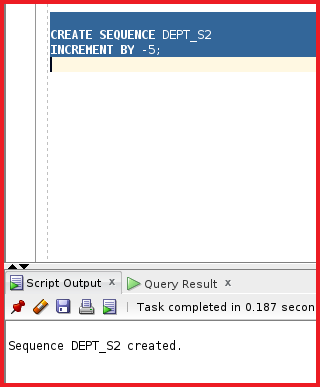So, the sequence is created. Let’s check the sequence details using the data dictionary USER_SEQUENCES.

select * from USER_SEQUENCES where SEQUENCE_NAME=’DEPT_S2’;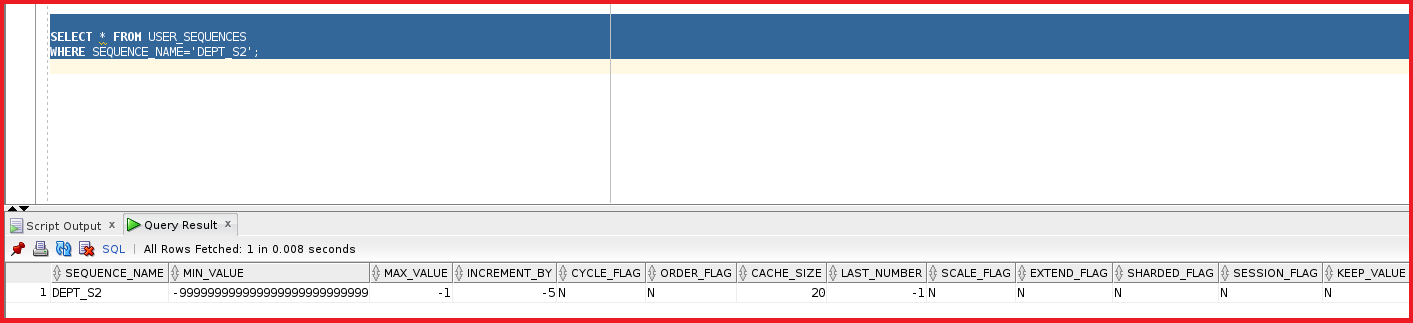We can see the sequence_name is DEPT_S2 and the min_Value is -9 and max_value is -1 and the increment by -5. In order to test this sequence let’s try to insert two records and see how this sequence works.

```INSERT INTO DEPT_TEST_S (DEPNO, DNAME) VALUES (DEPT_S2.NEXTVAL,'Marketing');
INSERT INTO DEPT_TEST_S (DEPNO, DNAME) VALUES (DEPT_S2.NEXTVAL,'Help Desk');```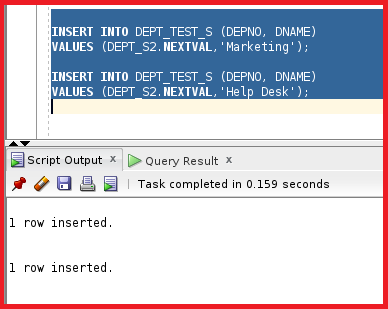We are using the same Marketing and Help Desk into the DNAME and the DEPNO will be the next value of the sequences. As the data is inserted let’s try to see the data in the table DEPT_TEST_S.

select * from DEPT_TEST_S;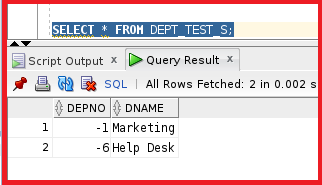We can see the sequences are going in the negative direction. The first record takes -1 and the second record is incremented by -5 and the value is -6. Now, let’s use the update statement for the table DEPT_TEST_S for the column DEPNO with the next value of the sequences.

UPDATE DEPT_TEST_S SET DEPNO=DEPT_S2.NEXTVAL;

Let’s execute this update statement.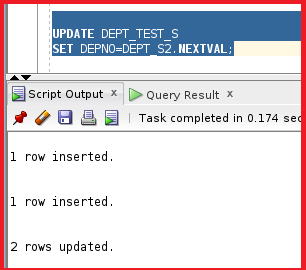So, after running the update statement it shows that two rows are updated. Let’s now check the table DEPT_TEST_S.

SELECT * FROM DEPT_TEST_S ORDER BY 1;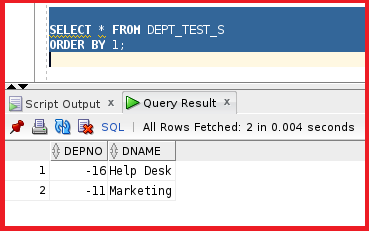So, the next value of the DEPNO is updated with the sequences incremented by -5.

##### Example 3:

Let’s have a look at another example of the sequences. We can create the default value as sequences in creating the table. In order to check this example let’s create a sequence EMP_S.

CREATE SEQUENCE EMP_S;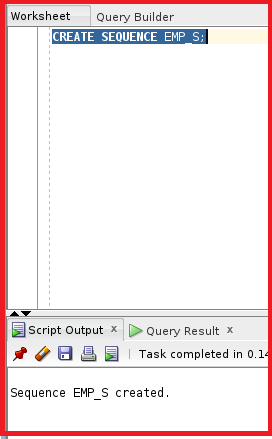So, the sequence is created. Let’s create a table called EM.

```CREATE TABLE EM
( EMPID NUMBER DEFAULT EMP_S.NEXTVAL PRIMARY KEY ,
NAME VARCHAR2(100),
DEPTNO NUMBER
);
```

This table consists of columns EMPID, NAME, and DEPTNO. The EMPID is a number and we can make this the default value which is sequences referring to the next value. This is also considered as the primary key. The other column is NAME with datatype as varchar2 and DEPTNO as number. Let’s try to create this table.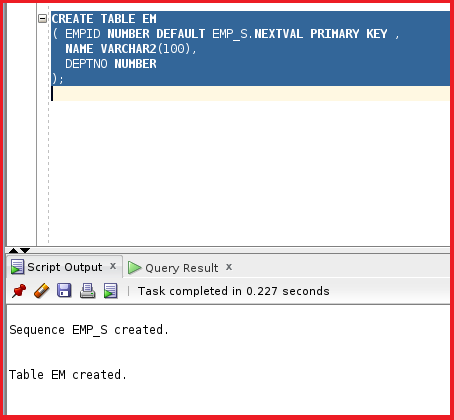So, the table is created. Let’s try to insert two records into the table EM.

```INSERT INTO EM (NAME ) VALUES ('JAMES');
INSERT INTO EM (NAME ) VALUES ('Mark');```

We are inserting only names into the table EM. The EMPID is taken as the default next value sequence. Let’s try to execute the insert statement.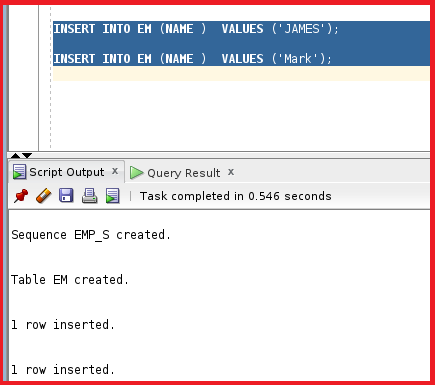So, the records are inserted. Let’s have a look at the table EM.

select * from EM;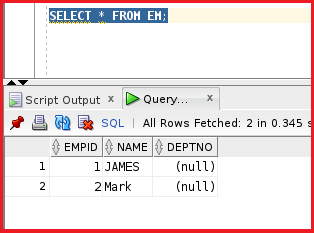We can see the EMPID as 1 for JAMES and 2 for MARK. This is taken by default which is came from sequences.

##### Example 4:

Let’s have a look at another example. We will try to learn about nextval and currval together in the script. Normally we use this to insert data into the master-detail table. We will learn how to alter the table and learn about the cycle. Let’s have a look at the example. Before that let’s delete the data from DEPT_TEST_S and EM tables.

delete from DEPT_TEST_S;

delete FROM EM;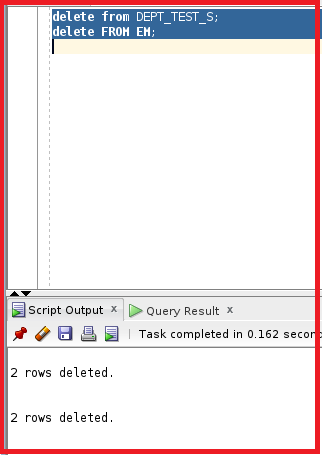So, the data inside the tables DEPT_TEST_S and EM tables are deleted. Now, we are reusing the sequence DEPT_S. For that, we need to drop and recreate the sequence DEPT_S.

drop sequence DEPT_S;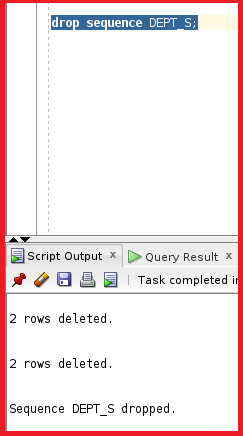So, the sequence is dropped. Let’s try to recreate the sequence.

CREATE SEQUENCE DEPT_S;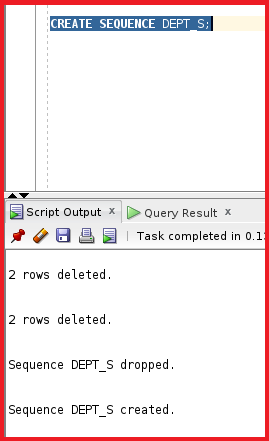So, the sequence is created again. The sequence is created with default values. Now we will try to learn about currval and nextval in inserting scripts. We will try to insert the department called support in the table DEPT_TEST_S using the sequence DEPT_S. Once the department is inserted into the table DEPT_TEST_S then insert 3 employees working with the department into the table EM. Let’s have a look at the statement.

```INSERT INTO DEPT_TEST_S (DEPNO, DNAME) VALUES (DEPT_S.NEXTVAL,'support');
INSERT INTO EM (NAME,DEPTNO )  VALUES ('ali',DEPT_S.CURRVAL);
INSERT INTO EM (NAME,DEPTNO )  VALUES ('ahmed',DEPT_S.currval);
INSERT INTO EM (NAME,DEPTNO )  VALUES ('samer',DEPT_S.currval);
```

So, we are inserting one record into the table DEPT_TEST_S and the sequence will start from 1. So, after inserting the record in the table DEPT_TEST_S the current value will be 1 in sequences. We will use the current value and insert that current value of sequences as the DEPTNO into the table EM. Let’s try to execute this insert statement.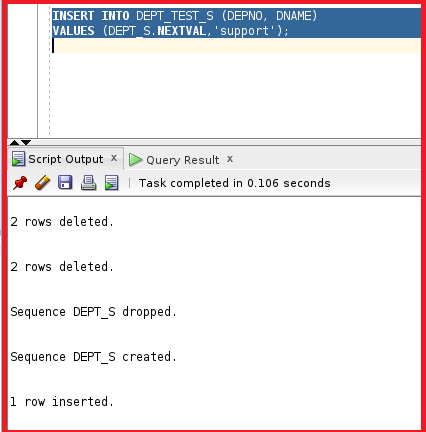The record is inserted into the table DEPT_TEST_S. Now, let’s execute the insert statement into the table EM.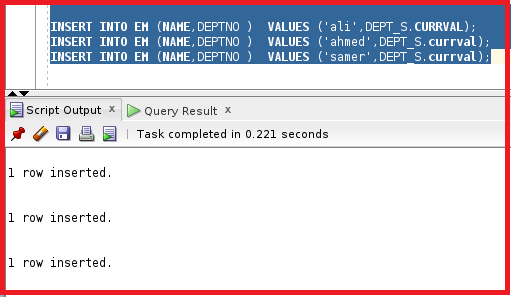So, the data is inserted into the tables DETP_TEST_S and EM. Let’s have a look at those tables.

select * from DEPT_TEST_S;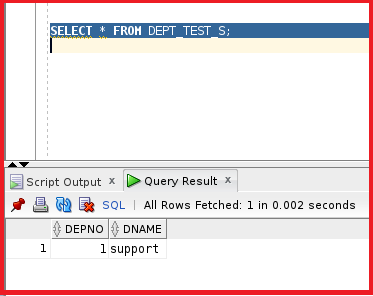We have depno as 1. Now let’s have a look at the table EM.

select * from EM;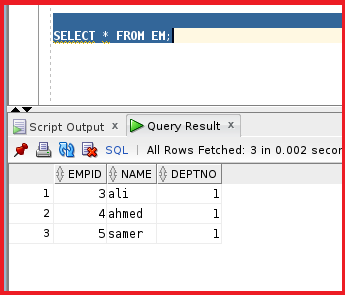We have three employees ali, ahmed, and samer with the same deptno which is the current value of the sequences. The EMPID is the default value taken by sequences.

##### Example 5:

Let’s now try to learn how to alter the sequences. To learn about altering the sequences. Let’s have a look at the sequence details of the sequence DEPT_s

SELECT * FROM USER_SEQUENCES WHERE SEQUENCE_NAME=’DEPT_S’;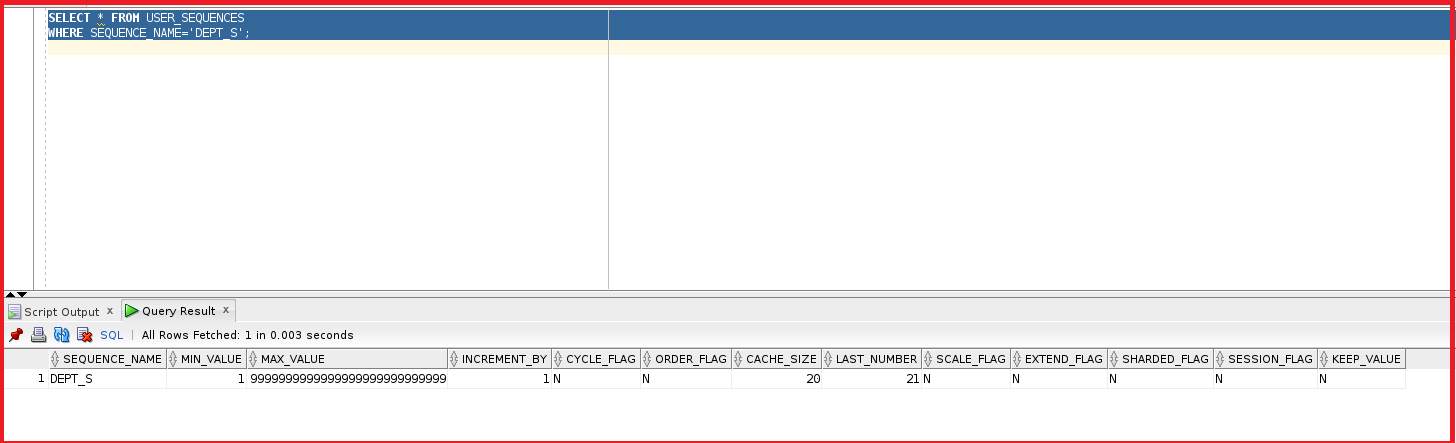We can see the minimum value is 1 and increment by 1 and the cache size is 20. Let’s try to make some changes to the sequences. We can make changes to the sequences using alter statement. Let’s try to increment the sequences by 100.

ALTER SEQUENCE DEPT_S INCREMENT BY 100;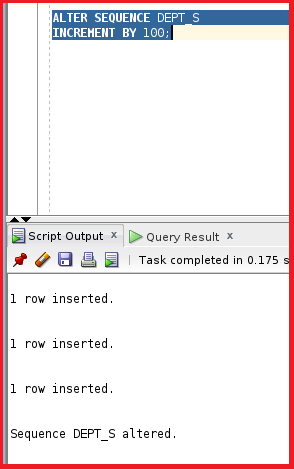So, the sequence is altered with increments of 100. Let’s try to check the details of the sequence now.

SELECT * FROM USER_SEQUENCES WHERE SEQUENCE_NAME=’DEPT_S’;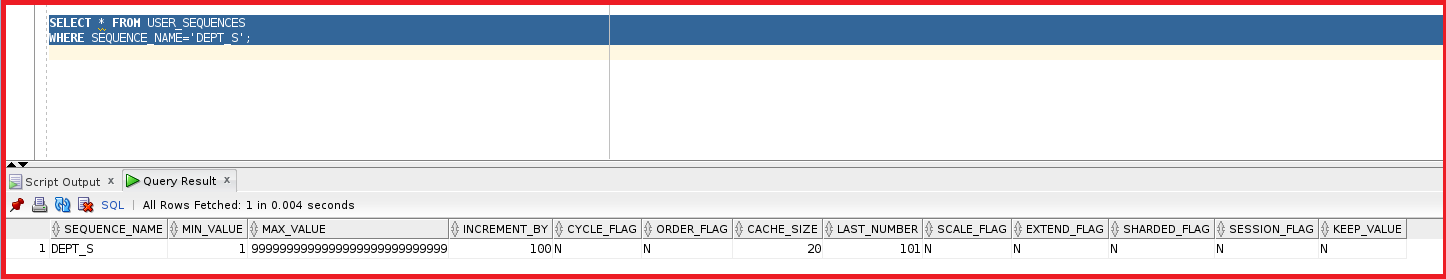We can see the increment by value is changed to 100. Now, let’s try to change the cache value to 30.

ALTER SEQUENCE DEPT_S CACHE 30;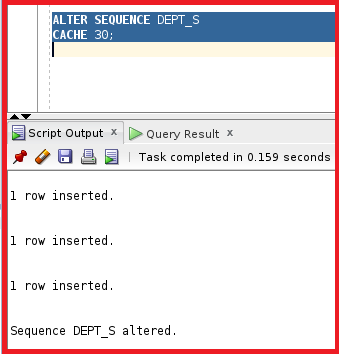Let’s try to change the maximum value to 9999.

ALTER SEQUENCE DEPT_S max_value 9999;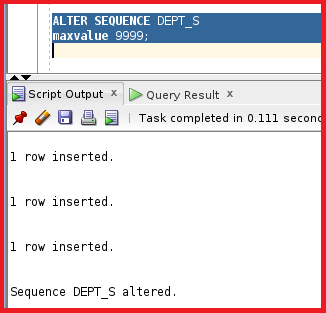So, we have changed the max value to 9999. Now, let’s have a look at the sequence details.

SELECT * FROM USER_SEQUENCES WHERE SEQUENCE_NAME=’DEPT_S’;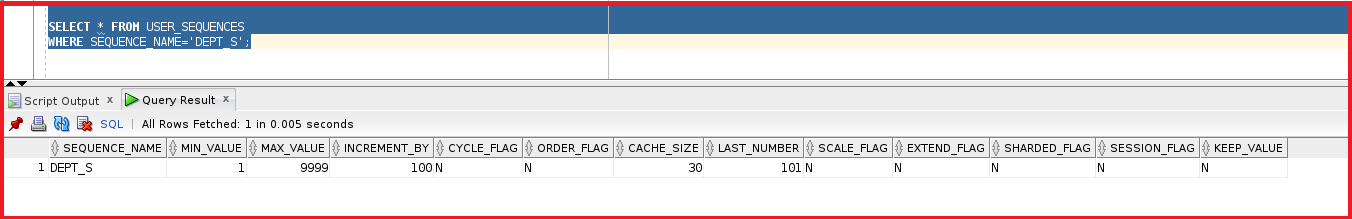We can see the details of the sequences where the cache_size is 30 and the max_Value is 9999. We cannot change the start with value once the sequence is created.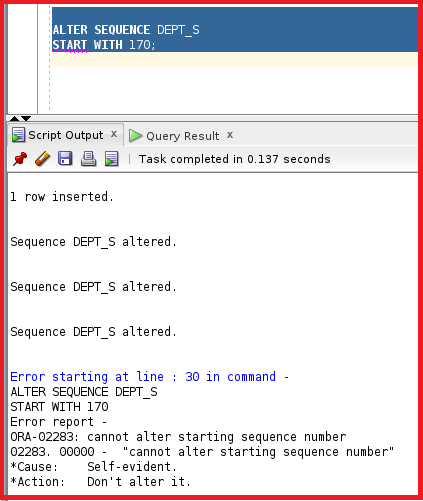We cannot alter the start with the parameter in sequence. So, now let’s try to learn about the cycle in sequences.

```CREATE SEQUENCE S_CYCLE
INCREMENT BY 1
MAXVALUE 5
nocache
CYCLE;
```

We have the sequence name as S_CYCLE which starts with 1 and the sequence will be incremented by 1 till the max value of 5. This sequence has no cache. The cycle indicates when the sequence reaches the max value then the sequence again starts with 1. Now, let’s try to create the sequence.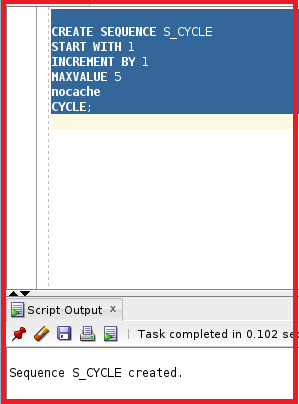So, we can see the S_CYCLE sequence is created. Now, let’s try to execute the select statement with nextval and see if the sequence works with the cycle.

select S_CYCLE.NEXTVAL from dual;

We can execute this 5 times and then for the next time the sequence will be reset to 1.

NEXTVAL=1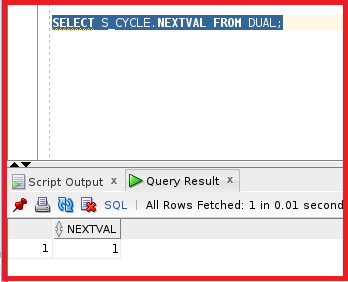NEXTVAL=2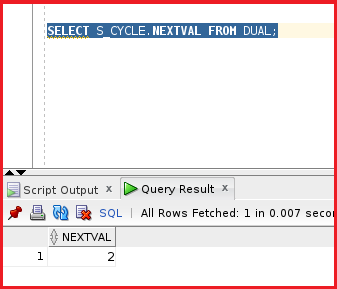NEXTVAL=3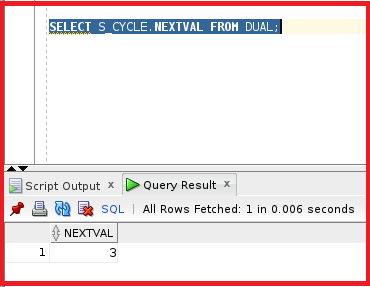NEXTVAL=4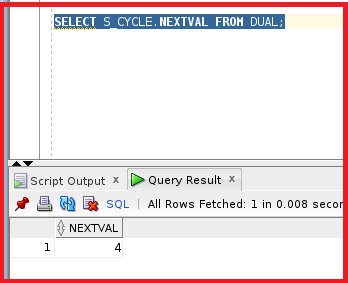NEXTVAL=5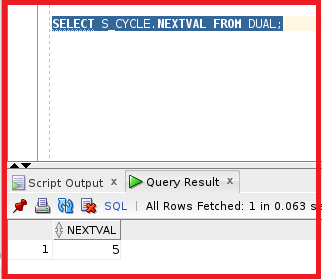So, the nextval is 5. If we try to run the select query again the nexval will be reset to 1. Let’s test that.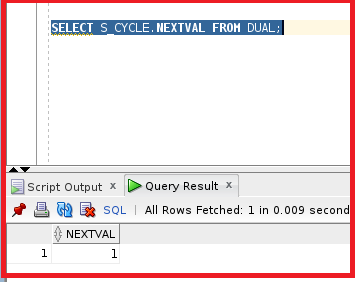So, these are a few examples, that we have used to test the Sequences in Oracle.

In the next article, I am going to discuss Synonyms in Oracle with Examples. Here, in this article, I try to explain Examples of Sequences in Oracle. I hope you enjoy this Examples on Sequences in Oracle article.